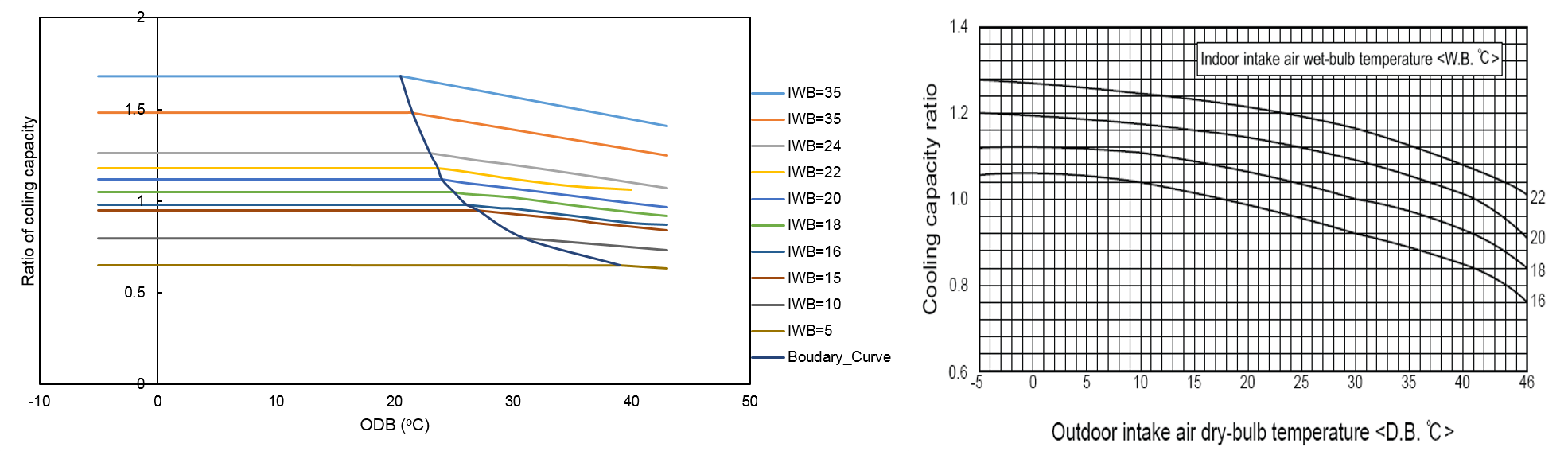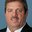Question-and-Answer Resource for the Building Energy Modeling Community
Get started with the Help page

VRF performance curve for non-flat low/high CapFT values

I have developed a performance curve for a VRF system (Left-hand side figure). My questions are;

1. In order to plot boundary curve (Cubic) I used Low temperature region regression equation to calculate CapFT from ODB and then plotted it as shown in the Left-hand side figure, this clearly gives a better fit. I am wondering, is this the right way or I am missing something?
2. If the above is correct and as I used low temperature region regression curve then what about when the low curves are not flat (e.g.Right-hand figure below)? In this case is it better to use table method and do we still need to do extrapolation? If we still need extrapolation for other temperature ranges then how to find the point between low and high regions?
3. What if pipe correction and Part-load performance datasets/curves are not available, is there a workaround to model VRF systems in this case?edit retag close merge delete

Sort by » oldest newest most voted1. This is the correct way to plot the boundary curve using the CAPFT results. However, the model still expects ODB as a function of IWB.
2. The high ODB temperature curves would be used when the ODB temperature is on the right side of the boundary curve. You would create a performance curve for the right side of the figure just as you did on the left side. The points between the low and high regions is the boundary curve you created in 1. above.
3. If specific performance aspects of VRF are not available you can used the data in the example file. Piping correction is not a large part of the answer but should still be included using your best judgment. Part-load performance is a large part of the answer yet using a curve similar to the example file is not that bad of an assumption. See this document for a description of how to create all the VRF performance curves.
more

@rraustad: Thanks for your answer, but in right hand side figure there is no distinct low and high temperature sides. How you would differentiate between these regions? P.S: The curve in left hand side figure is for another system not the one on right hand side.

I meant the right-hand side of the left figure. If you used the right figure, you would only have 1 performance curve and not need the boundary curve. If you use the boundary curve, you also create two performance curves for the data to the left and right of the boundary curve.

Thanks, I will have a look.

@rraustad: I was wondering, if the low temperature curves are not straight in left hand side figure, then which equation to use for converting boudary curve temperature to CAPFT (i.e. low tempearture curves or high temperature curves). In the above left hand side figure it was easy as the low temperature curves were straight.

Just enter the IWB and ODB (where the left and right curves meet) into the CAPFT performance curve and then plot CAPFT vs ODB. Even if the curve is not straight, the curve is still a function of IWB and ODB, so if it curves downward, then that curve still represents a single IWB temperature.

Here is the document about generating VRF performance curve:

more

Thanks, I have it already. :)